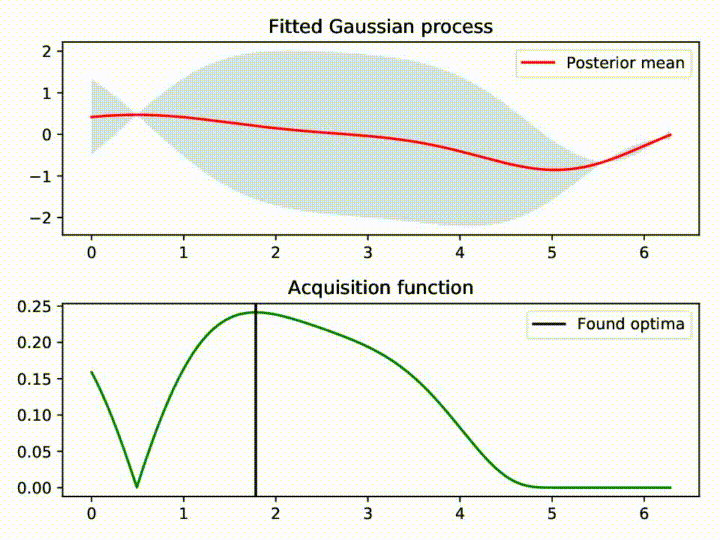# pyGPGO: Bayesian Optimization for PythonpyGPGO is a simple and modular Python (>3.5) package for bayesian optimization.

Bayesian optimization is a framework that can be used in situations where:

• Your objective function may not have a closed form. (e.g. the result of a simulation)
• No gradient information is available.
• Function evaluations may be noisy.
• Evaluations are expensive (time/cost-wise)

### Installation

Retrieve the latest stable release from pyPI:

``pip install pyGPGO``

Or if you're feeling adventurous, retrieve it from this repo,

``pip install git+https://github.com/hawk31/pyGPGO``

### Dependencies

• Typical Python scientific stuff: `numpy`, `scipy`.
• `joblib`, used for parallel computation
• `scikit-learn`, for other surrogates different from Gaussian Processes.
• `pyMC3`, for integrated acquisition functions and MCMC inference.
• `theano` for GPU processing.

All dependencies are automatically taken care for in the requirements file.

### Features

• Different surrogate models: Gaussian Processes, Student-t Processes, Random Forests, Gradient Boosting Machines.
• Type II Maximum-Likelihood of covariance function hyperparameters.
• MCMC sampling for full-Bayesian inference of hyperparameters (via `pyMC3`).
• Integrated acquisition functions

### A small example!

The user only has to define a function to maximize and a dictionary specifying input space.

``````import numpy as np
from pyGPGO.covfunc import matern32
from pyGPGO.acquisition import Acquisition
from pyGPGO.surrogates.GaussianProcess import GaussianProcess
from pyGPGO.GPGO import GPGO

def f(x, y):
# Franke's function (https://www.mathworks.com/help/curvefit/franke.html)
one = 0.75 * np.exp(-(9 * x - 2) ** 2 / 4 - (9 * y - 2) ** 2 / 4)
two = 0.75 * np.exp(-(9 * x + 1) ** 2/ 49 - (9 * y + 1) / 10)
three = 0.5 * np.exp(-(9 * x - 7) ** 2 / 4 - (9 * y -3) ** 2 / 4)
four = 0.25 * np.exp(-(9 * x - 4) ** 2 - (9 * y - 7) ** 2)
return one + two + three - four

cov = matern32()
gp = GaussianProcess(cov)
acq = Acquisition(mode='ExpectedImprovement')
param = {'x': ('cont', [0, 1]),
'y': ('cont', [0, 1])}

np.random.seed(1337)
gpgo = GPGO(gp, acq, f, param)
gpgo.run(max_iter=10)
``````

Check the `tutorials` and `examples` folders for more ideas on how to use the software.